Next: Conclusions to Chapter 3 Up: Criticism of the relativistic experiments Previous: GRT experiments   Contents

## Once again on light speed

The notion of "velocity" is clearly determined (remember police at way), and only for "the secret agent 007 - light" there exist many "passports" (according to relativists): some "Great" constant (for "a relativistic oath"); coordinate velocity (in this case relativists cannot to hide the necessity of "blasphemous" term c+v in any way) - but what can be "taken" from it; phase velocity (with it land-surveyors work , opticians calculate microscopes and telescopes with it, astronomers calculate refraction with it, etc.); group velocity (which was "with regret" introduced by Rayleigh and which is almost not used by practical workers, but which is often declared as "true" by relativists, if it does "accidentally" not turn out negative, or more than the constant nominated by them themselves). Sheer "a card-sharping with three glasses at a railway station building": have guessed right or not?

Though the problem of light speed has been considered above, we shall here formulate more clearly the law of velocity addition for a light signal (for the corpuscular and wave models of light) in the example of one-dimensional motion. Let the axis be directed from the source to the receiver. Let the source at distance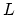from the receiver to emit a light beam having some frequency characteristic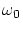. Then two situations are possible:
1) Irrespective of the nature of light, when the receiver moves at speed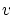relative to the source, the signal reception rate (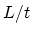) will be determined by the geometrical sum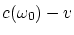, and the frequency of received light will be determined by the simplest classical Doppler law: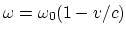. The question - what local velocity (all measurements are made inside the receiver of the fixed configuration) will be recorded by the receiver - is completely different: this quantity can depend on the nature of light (a wave? a point particle? a particle with inner degrees of freedom?), on the receiver design, on frequency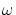, etc.
2) When the signal source moves at speed, the result depends on the nature of light. If light represents a flux of particles, then we obtain again the classical linear law of velocity addition: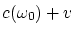. If light represents a wave, we actually deal with the addition of translational and oscillatory motions, and the theorist cannot write down the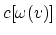dependence and the Doppler law in the explicit form. For the value of velocity, we can find, in principle, the linkage with characteristics of the "medium of propagation". Recall, for example, that the speed of sound in gases can be expressed in terms of the following quantities: the molecular weight of the gas, temperature, adiabatic index. For rigid bodies, the longitudinal and transverse speeds of sound are expressed in terms of density, Young's modulus and Poisson's coefficient; for liquids it is necessary to know some empirical factors. One of the possible hypotheses on the propagation rate for light in vacuum will be presented in Appendixes, where the light propagation process will be supposed to be mainly influenced by virtual electron-positron pairs. As far as the frequency is concerned, we find that it will be determined by the Doppler law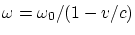within the limit of small oscillations only. In the case of arbitrary distances, directions of motion, arbitrary fields, possible presence of ether or of an inner structure of light (with additional degrees of freedom), essentially all dependencies can be complicated. Thus, in the general case, the determinations of the law of velocity addition, and the determination of light speed (again - not local, inside the receiver, but in vacuum between the source and receiver!) and the Doppler law, are the prerogative of experiment.Next: Conclusions to Chapter 3 Up: Criticism of the relativistic experiments Previous: GRT experiments   Contents
Sergey N. Arteha